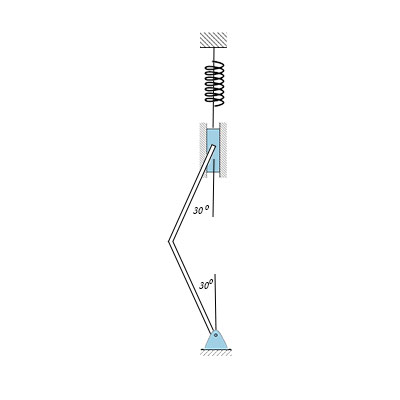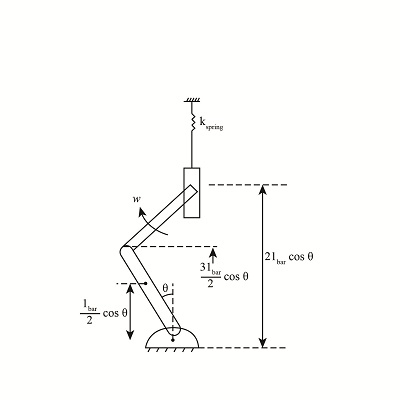Each bar has a mass of 8 kg and a length of 1 m. The spring is k=100 N/m and the spring...

Question:

Each bar has a mass of {eq}8 kg {/eq} and a length of {eq}1 m. {/eq} The spring is {eq}k=100 N/m {/eq} and the spring unstretched when {eq}\theta = 0 {/eq}. If the system is released from rest with the bars of the bars of the vertical what is the magnitude of the angular velocity of the bars with {eq}\theta = 30^o. {/eq}Law of Conservation of Energy:

A system posses motion from rest, then, according to the law of conservation of energy, the summation of all the energy at the rest condition is equal to the summation of all the energy at the final condition.

Given data:

• The value of the mass of the bar is {eq}{m_{bar}} = 8\;{\rm{kg}} {/eq}
• The length of the bar is {eq}{l_{bar}} = 1\;{\rm{m}} {/eq}
• The value of the angle at the initial condition is {eq}{\theta _i} = 0^\circ {/eq}
• The value of the angle at the final condition is {eq}{\theta _f} = 30^\circ {/eq}
• The value of the stiffness of the spring is {eq}{k_{spring}} = 100\;{\rm{N/m}} {/eq}The expression for the stretch length of the spring is

{eq}x = 2{l_{bar}}\left( {1 - \cos \theta } \right) {/eq}

The expression for the total potential energy at the initial condition is

{eq}\begin{align*} P.{E_{tota{l_{initial}}}} &= P.{E_{spring}} + P.{E_{bar}}\\ &= \dfrac{1}{2}{k_{spring}}{x_s}^2 + \left( {{m_{bar}}g\left( {\dfrac{{{l_{bar}}}}{2}\cos \theta } \right) + {m_{bar}}g\left( {\dfrac{{3{l_{bar}}}}{2}\cos \theta } \right)} \right)\\ &= \dfrac{1}{2}{k_{spring}}{\left( {2{l_{bar}}\left( {1 - \cos \theta } \right)} \right)^2} + 2{m_{bar}}g{l_{bar}}\cos \theta \end{align*} {/eq}

Substitute the value in the above equation:

{eq}\begin{align*} P.{E_{tota{l_{initial}}}} &= \dfrac{1}{2}\left( {100} \right){\left( {2 \times 1\left( {1 - \cos 0} \right)} \right)^2} + 2\left( 8 \right)g\left( 1 \right)\cos 0\\ &= 156.96\;{\rm{N}}{\rm{.m}} \end{align*} {/eq}

The expression for the final total potential energy is

{eq}\begin{align*} P.{E_{tota{l_{final}}}} &= \dfrac{1}{2}{k_{spring}}{x^2} + 2{m_{bar}}{l_{bar}}\cos \theta \\ P.{E_{tota{l_{final}}}} &= \dfrac{1}{2}{k_{spring}}{\left( {2{l_{bar}}\left( {1 - \cos \theta } \right)} \right)^2} + 2{m_{bar}}{l_{bar}}\cos \theta \end{align*} {/eq}

Substitute the value in the above equation:

{eq}\begin{align*} P.{E_{tota{l_{final}}}} &= \dfrac{1}{2}\left( {100} \right){\left( {2 \times 1\left( {1 - \cos 30} \right)} \right)^2} + 2 \times 8 \times 1\cos 30\\ & = 139.6\;{\rm{N}}{\rm{.m}} \end{align*} {/eq}

The expression for the total kinetic energy at the final condition

{eq}\begin{align*} K.{E_{tota{l_{final}}}} &= \dfrac{1}{2}{I_{inerti{a_{AB}}}}{\omega ^2} + \dfrac{1}{2}{I_{inerti{a_{BC}}}}{\omega ^2} + \dfrac{1}{2}{m_{bar}}{v_g}^2\\ &= \dfrac{1}{2}\left( {\dfrac{{{m_{bar}}{l_{bar}}^2}}{3}} \right){\omega ^2} + \dfrac{1}{2}\left( {\dfrac{{{m_{bar}}{l_{bar}}^2}}{{12}}} \right){\omega ^2} + \dfrac{1}{2}{m_{bar}}\left( {\dfrac{{{\omega ^2}{l_{bar}}^2}}{4}\left( {1 + {{\sin }^2}\theta } \right)} \right) \end{align*} {/eq}

Substitute the value in the above equation:

{eq}\begin{align*} K.{E_{tota{l_{final}}}} &= \dfrac{1}{2}\left( {\dfrac{{8 \times {1^2}}}{3}} \right){\omega ^2} + \dfrac{1}{2}\left( {\dfrac{{8 \times {1^2}}}{{12}}} \right){\omega ^2} + \dfrac{1}{2} \times 8\left( {\dfrac{{{\omega ^2} \times {1^2}}}{4}\left( {1 + {{\sin }^2}30} \right)} \right)\\ &= 4.66{\omega ^2}\;{\rm{N}}{\rm{.m}} \end{align*} {/eq}

The expression for the law of conservation of energy is

{eq}K.{E_{initial}} + P.{E_{initial}} = K.{E_{final}} + P.{E_{final}} {/eq}

Initially, the system is at rest, so the kinetic energy at the initial condition is zero. Substitute the value in the above equation:

{eq}\begin{align*} 0 + 156.96 &= 4.66{\omega ^2} + 139.6\\ \omega &= 1.93\;{\rm{rad/s}} \end{align*} {/eq}

Thus, the value of the angular velocity is {eq}1.93\;{\rm{rad/s}} {/eq}.